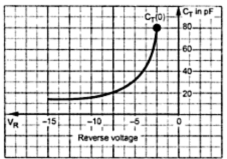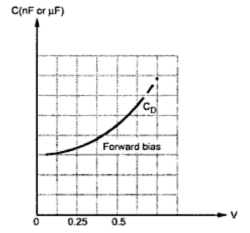### Junction Capacitances

Depending upon the biasing condition, two types of capacitive effects exist in the diodes. These are,
1) Transition capacitance (CT) is reversed biased condition.
2) Diffusion capacitance (CD) is forward biased condition.
1.1 Transition Capacitance (CT or Cpn)
When a diode is reversed biased, the width of the depletion region increases. So there are more positive and negative charges present in the depletion region.Fig. 1

Due to this, the p-region and n-region act like the plates of capacitor while the depletion region acts like dielectric. Thus there exists a capacitance at the p-n junction called transition capacitance, barrier capacitance or depletion region capacitance. It is denoted as CT.
Mathematically it is given by the expression,
where       ε  = permittivity of semiconductor = εo εr
εo = 1/(36π x109 ) = 8.849 x 10-12   F/m
εr = Relative permittivity of semiconductor = 16 for Ge, 12 for Si
A = area of cross section
W = width of depletion region
As the reverse biased applied to the diode increases, the width of the depletion region (W) increases. Thus the transition capacitance CT decreases. In short, the capacitance can be controlled by the applied voltage. The variation of  CT with respect to the applied reverse bias voltage is shown in the Fig. 2.Fig. 2

As reverse voltage is negative, graph is shown in the second quadrant. For a particular diode shown, CT varies from 80 pF to less than 5 pF as VR changes from 2 V to 15 V.
Note : The transition capacitance is also called depletion layer capacitance and denoted as Cpn.
1.2 Diffusion Capacitance (CD)
During forward biased condition, an another capacitance comes into existence called diffusion capacitance or storage capacitance, denoted as CD.
In forward biased condition, the width of the depletion region decreases and holes from p side get diffused in n side while electrons from n side move into the p-side. As the applied voltage increases, concentration of injected charged particles increases. This rate of change of the injected charge with applied voltage is defined as a capacitance called diffusion capacitance.
The diffusion capacitance can be determined by the expression
where τ = mean life time for holes.
So diffusion capacitance is proportional to the current. For forward biased condition, the value of diffusion capacitance is of the order of nano farads to micro farads while transition capacitance is of the order of pico farads. So CD is much larger than CT.
However in forward biased condition, CD appears in parallel with the forward resistance which is very very small. Hence the time constant which is function of product of the forward resistance and  CD is also very small for ordinary signals.
Note : Hence for normal signals CD has no practical significant but for fast signals CD must be considered.
The graph of CD against the applied forward voltage is shown in the Fig. 3.Fig. 3  Diffusion capacitance versus applied forward biased voltage